3.2. Synchrotron emission

The first experimental evidence of the existence of large-scale magnetic fields in external galaxies came, historically, from the synchrotron emission of Crab nebula . The emissivity formula (i.e. the energy emitted from a unit volume, per unit time, per unit frequency interval and per unit solid angle) for the synchrotron is a function of Band and of the relativistic electron density, namely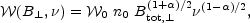(3.4)

whereis the frequency,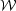0 is a proportionality constant depending only upon the spectral indexwhich also determines the (isotropic) relativistic electron number density distribution for electrons with energies in the range dE: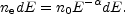(3.5)

A useful estimate for the maximal emission from electrons of energy E is given by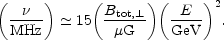(3.6)

In generic terms the synchrotron emission is then sensitive to the total transverse magnetic field. In oversimplified terms the measurement proceeds in three steps:

• estimate of the emission intensity;
• from the estimate of the emission intensity, defining as L the typical size of the source, the quantity Btot,(+1)/20L can be deduced;

• the magnetic field can then be obtained by specifying the electron density distribution of the source.

While the second step is rather well defined (can indeed be determined from the observed emission spectrum (6)) the third step has to be achieved in a model-dependent way. The relativistic electron density is sometimes estimated using equipartition, i.e. the idea that magnetic and kinetic energy densities may be, after all, comparable. Equipartition is not always an empirical evidence, but can certainly be used as a working hypothesis which may or may not be realized in the system under consideration. For instance equipartition probably holds for the Milky Way but it does not seem to be valid in the Magellanic Clouds . The average equipartition field strengths in galaxies ranges from the 4µ G of M33 up to the 19µ G of NGC2276 [27, 28].

In order to illustrate the synchrotron emission take for instance the MW. Away from the region of the galactic plane synchrotron emission is the dominant signal of the galaxy for frequencies below 5 GHz. Between 1.4 and 5 GHz the spectral dependence is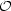(-0.9). It should be noticed that the spectral index of synchrotron emission may change in different frequency ranges and also in different regions of the sky due to the variation of the magnetic field . This aspect is crucial in the experimental analysis of CMB anisotropy experiments in the GHz region. In fact, in the context of CMB studies the synchrotron is a foreground which should be appropriately subtracted. The way this is done is by extrapolation of lower frequency measurements. The problem of proper subtraction of synchrotron foreground is particularly acute in the case of the PLANCK mission (7)

The synchrotron has an intrinsic polarization which can give the orientation of the magnetic field, but not the specific sign of the orientation vector. The strength of synchrotron polarization is proportional to |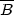/ Btot,|2, i.e. the ratio between the magnetic energy densities of the uniform and total magnetic field. Thus, according to Eq. (3.1), the synchrotron polarization is sensitive to the random component of the large-scale magnetic field.

6 Typical galactic values ofare between 2.4 and 2.8. An important caveat to this statement, relevant for foreground extraction (for instance in studies of CMB anisotropies) is that the synchrotron spectrum may have a break. Back.

7 The satellite mission PLANCK EXPLORER will provide, after 2008, full sky maps in nine frequency channels ranging from 30 to 900 GHz. Back.